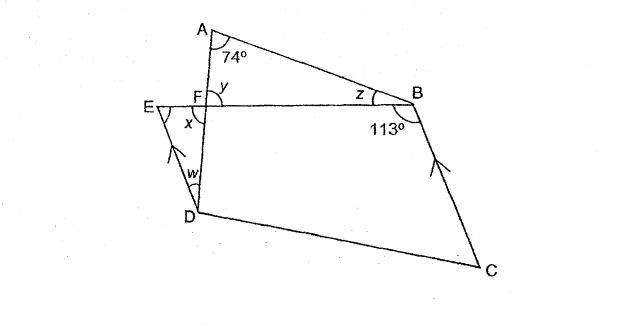Question 1 of 25

In the figure below, EBCD is a trapezium. ED is parallel to BC. $\angle$FAB = 74$^\circ$ and $\angle$EBC = 113$^\circ$. Find the sum of $\angle$w, $\angle$x, $\angle$y,and $\angle$z.A
213$^\circ$
B
215$^\circ$
C
217$^\circ$
D
219$^\circ$
E
None of the above